• Submissions# Electrochemical Behavior of Pb/Pb-MnO2 Composite Coated Anodes Used in Zinc Electrowining Industry

1 Faculty of Materials Engineering, Sahand University of Technology, Iran

2 Faculty of Materials Engineering, Zanjan University, Iran

3 Faculty of chemistry, Tabriz University, Iran

*Corresponding author: Parvini Ahmadi Naghi, Faculty of Materials Engineering, Sahand University of Technology, Tabriz, Iran

Submission: May 15, 2018; Published: June 25, 2018

DOI: 10.31031/RDMS.2018.06.000644ISSN: 2576-8840
Volume6 Issue4

#### Abstract

In this study, corrosion behavior of Pb-MnO2 composite coatings were developed on the pure lead anodes with 5, 10, 15 and 20 MnO2 (wt-%) in acidic zinc sulphate electrolyte were investigated. The method of coating was cold spraying technology. The electro catalytic activity towards oxygen evolution reaction (OER) and corrosion resistance of samples were evaluated using potentiodynamic polarization and electrochemical impedance spectroscopy (EIS) methods. The results point to that Pb-15MnO2 (wt-%) composite coating anode had the lowest corrosion activity among the other examined anodes. Also, for investigating the mechanism of corrosion electrochemical noise techniques method (ZRA) were done. The results indicated that the Pb-15MnO2 (wt-%) has uniform corrosion approximately but the other coatings showed the pitting corrosion.

Keywords: Pb-MnO2 Composite coating; EIS; OER; Electrochemical noise techniques method (ZRA); Pitting corrosion

#### Introduction

Pb and Pb alloys are used as anodes in electro winning of different metals such as zinc [1,2]. However these anodes have some disadvantages such as Pb contamination on the cathode and high oxygen evolution over potential (OER), so composite anodes have been noticeable for some researcher recently [3-6]. Composite anodes have been developed to combine the advantages of conventional anodes in electro winning, such as lead-based alloys and DSA. Composite anodes consist of active particles of metal oxide dispersed in a matrix composed of lead dioxide and lead . It is possible to use Sabatier principle to compare the catalytic properties of various oxides for the reaction of oxygen evolution. Based on this method, the best catalytic performance is observed when the interface reaction is neither too weak nor too strong . If the oxide has more stable oxide state, oxygen mediators are strongly absorbed to its surface and hardly separated from it; this will help to block the surface. On the other hand, if the interaction is poor, the intermediates fail to bind to the oxidation surface, and therefore no reaction occurs . Different composites materials such as Co-Ti nano particles , P-(PbO2-MnO2) and (P-PbO2)-MnO2  have been suggested for electro winning anodes. Spinal-type oxides, especially cobaltite (CO3O4, NiCO2O4) exhibit high electro catalytic activity in the reaction of oxygen evolution .

MnO2 can be considered as an active oxide for OER because of its high catalytic activity toward OER [9,13]. The catalytic activity of this oxide being a Rutile type has already been reported for the reaction of oxygen evolution in acid and alkaline solutions [7,14-17]. Pb-MnO2 composite anodes have been prepared by co-precipitation of MnO2 particles on lead surface [18-20]. MnO2 particles showed positive effects on the catalytic activity of the lead anode in the reaction of oxygen evolution during anodic polarization tests.

Pb-Pb-MnO2 composite anodes had been prepared as containing different MnO2 contents and using different structures of MnO2 . Short-term results of the composite anodes (6 hours) indicated that the MnO2-containing composite anodes have better catalytic activity for OER than the Pb-Ag anode. Powder metallurgy process has been recently considered by researchers for producing the Pb-MnO2 composite anodes. The results showed that the anodic layers formed on Pb-MnO2 composite anodes exhibit excellent corrosion resistance in sulphuric acid electrolyte compared to Pb- Ag anodes. This phenomenon is due to the more uniform, thinner, and denser anodic layer formed in Pb-MnO2 composite anodes in acidic electrolyte solution [21-23].

The amount of activity of the electrodes for a reaction such as oxygen liberation is a function of surface area, surface imperfections, and surface morphology. Therefore, the preparation of composite coatings with different MnO2 percentages creates different surface properties. In this study, corrosion behavior of the different Pb- MnO2 composite coating developed on virgin pure Pb anodes by cold spray method has been studied. The aim of this study was determination of the best amount of MnO2 as electro catalyst materials in Pb-MnO2 composite anodes for corrosion activity in zinc electro winning industry. The concentration was on studying the effect of MnO2-%wt on corrosion mechanisms and corrosion resistance of coatings which is not considered by many researchers.

#### Experimental Section

For this study different Pb-MnO2 composite coating were applied on pure Pb anodes with amount of 5, 10, 15 and 20 (wt-%) MnO2. Coating method was cold spraying process. The electrochemical behavior of coated samples was performed by a computer-controlled Ivium potentiostat to compare the corrosion behavior of the coatings. Analysis was carried out in industrial zinc sulphate electro winning solution at 38 °C. Coated specimens, saturated Kalomel electrodes (SCE) and platinum were used as working, reference and counter electrodes, respectively. At first, electrochemical impedance spectroscopy (EIS) method were applied on specimens in the frequency range 100kHz to 10mHz with amplitude of 5mV (SCE) around the open circuit potential. After EIS test, potantiodynamic polarization experiments were performed in the potential range of-500-1000mV (SCE), around oxygen evolution over potential with a step of 10 mV and the scan rate of 0.002V(SCE)/s. Finally electrochemical noise techniques method (ZRA) was use for studying the corrosion behavior of the samples. The basis of this technique is to measure disturbances in flow and potential of two similar samples.

#### Results and Discustion

The potentiodynamic characteristics of the different coatings measured in the sulfate acid solution are plotted in Figure 1. Corrosion parameters obtained from polarization charts are presented in Table 1 using the tofel least squares matching method. The results are shown a significant reduction in the corrosion current density (Icorr) of Pb-15MnO2 (wt-%) coating and the increase in linear polarization (RP) of this sample. In the Pb-5% MnO2, Pb-10% MnO2 and Pb-20% MnO2, due to the presence of micro-cracks and cavities, the connection paths are formed between the electrolyte environment and the lead substrate. This also intensifies the pitting corrosion compared with the pure lead substrate.

Figure 1:The potatiodynamic polarization graphs related to Pb-5MnO2 (wt-%), Pb-10MnO2 (wt-%), Pb-15MnO2 (wt-%) and Pb- 20MnO2 (wt-%) coatings in industrial zinc sulfate solution.Table 1: Electrochemical corrosion parameters for Pb-5MnO2 (wt-%), Pb-10MnO2 (wt-%), Pb-15MnO2 (wt-%) and Pb-20MnO2 (wt-%) in industrial zinc sulfate.In equivalent circuit Rct is the charge transfer resistance, Rs is the electrolyte penetration resistance and Q is the ideal capacitor or constant phase element (CPE). Due to the fact that the double layer created between the electrode and the electrolyte surface are not exactly parallel, and also the coated electrode layer is rough and heterogeneous, the constant phase element is used instead of the ideal capacitor. By calculating the total circuit impedance, equation (1) is obtained: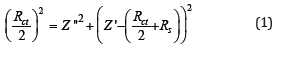Which is an equation of a circle with radius of Rct/2 and the distance of the center of the circle from the origin of the coordinates are Rct/2+Rs. Therefore, it can be seen that the corrosion resistance of the coating has increased with increasing the diameter of the semicircle in the Nyquist chart. Therefore, according to Figure 2 the Pb-15MnO2 (wt-%) coating has the highest corrosion resistance. Also the Pb-10MnO2 (wt-%) coating has a higher corrosion resistance than the Pb-20MnO2 (wt-%) coating (Figure 3).

Figure 2:The Nyquist diagram for Pb-10MnO2 (wt-%), Pb-15MnO2 (wt-%) and pb-20MnO2 (wt-%) coatings in industrial zinc sulfate solution.Figure 3:The electrochemical equivalent circuit used for equating Nyquist diagrams.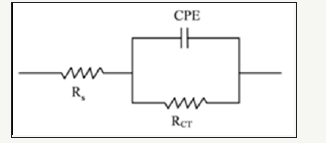Figure 4:Comparison of the equilibrated Bode curves with the actual sample for Pb-10MnO2 (wt-%) composite coating.Figure 5:Comparison of the equilibrated Bode curves with the actual sample for Pb-15MnO2 (wt-%) composite coating..Simulation of samples measured with electrochemical equivalent circuit was done by Zsimps software. The accuracy of the equivalent curves compared to the actual curves is shown in Figure 4-6. As can be seen, the equilibrium has been able to fit well with the Bode diagrams. The values of the parameters obtained from this equivalency are given in Table 2. These values illustrate the higher corrosion resistance of Pb-15MnO2 (wt-%) than the other samples obviously. This increase is about 30% higher than the Pb- 10MnO2 (wt-%) sample and 50% higher than the Pb-20MnO2 (wt- %) sample.

Figure 6:Comparison of the equilibrated Bode curves with the actual sample for Pb-20MnO2 (wt-%) composite coating.Table 2: The values of the parameters obtained from Nyquist diagrams equivalency.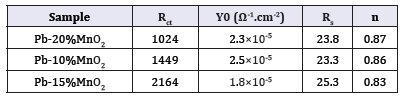The Bode diagrams obtained from the EIS test for Pb-10MnO2 (wt-%), Pb-15MnO2 (wt-%) and Pb-20MnO2 (wt-%) have shown in Figure 7-9 respectively. Each of these graphs has two distinct curves, one of which is the phase changes in terms of the logarithm of frequency (dome shape), and the other is the logarithm of the impedance in terms of the logarithm of frequency. As far as the maximum point of this chart is found to the right the more, it indicates the higher protection properties of the coating against corrosion. Therefore, it can be concluded that the Pb-15MnO2 (wt- %) coating has better protective properties than the other two (Figure 10).

Figure 7:The Bode diagrams obtained from the EIS test for Pb-10MnO2 (wt-%).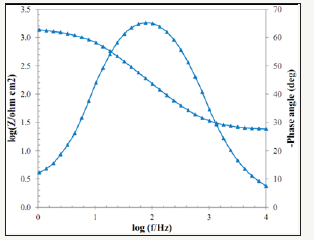Figure 8:The Bode diagrams obtained from the EIS test for Pb-15MnO2 (wt-%).Figure 9:The Bode diagrams obtained from the EIS test for Pb-20MnO2 (wt-%).Figure 10:The comparison between the phase angles in terms of the frequency diagrams of the Pb-10MnO2 (wt-%), Pb-10MnO2 (wt-%) and Pb-10MnO2 (wt-%).Figure 11:A schematic of logarithmic changes in impedance in terms of frequency logarithm.The other part of Bode diagram (the logarithm of the impedance in terms of the logarithm of frequency) is shown in Figure 11 schematically. The right arrow of the chart (impedance at the highest frequency) shows the soluble resistance (Rs), because according to the electrochemical equivalent circuit, if the very high sinusoidal frequency is entered into the circuit, the capacitor (CPE) is practically charge and discharge so it acts like a short circuit. Therefore, the current passes through the segment of the capacitor completely. So, the only current resistance is the soluble resistance (Rs). The left arrow (impedance at the lowest frequency) in Figure 11 represents the sum of the solution resistance and the charge transfer resistance. Due to the fact that the solution resistance is negligible in compare to the charge transfer resistance of the coating, so this value can be attributed. If assumed that in the electrochemical equivalent circuit the frequency is so low that it is virtually possible to assume that the current is DC (not sinusoidal), so the capacitor is charged in the first micro-seconds and no permits the current passes through its part. Therefore, solution resistance and the charge transfer resistance of the coating set as series. Therefore, the higher the left side of the graph, the more resistance it is to the coating . These values are compared in Figure 12 for various coatings. As shown in Figure 12, these values also confirm the results of the Nyquist diagrams, and the Pb-15MnO2 (wt-%) sample has a higher resistance than the other two.

Figure 12:Comparison of logarithmic impedance variations in terms of frequency logarithm for Pb-20MnO2 (wt-%), Pb-10MnO2 (wt-%) and Pb-15MnO2 (wt-%) coated samples.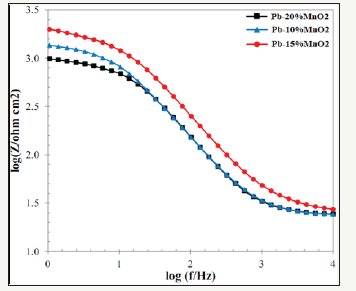Another basic parameter according to Bode diagram is the capacitive region and the resistance zone. As shown in Figure 11, the impedance-frequency curve is divided into two parts. A section with a diagonal arrow, called the capacitive region and the part shown with the horizontal arrow, is called the resistance zone. It is proved that increasing the size in the capacitive region and decreasing in the resistance zone indicates better resistance properties of coating against the entry of the electrolyte and corrosive agents and to reach the substrate surface. Figure 12 indicate that the Pb-15MnO2 (wt-%) coating has the highest value for the capacitive region, which shows the most corrosion resistance of this coating among the others .

Another important parameter of the impedance-frequency curve is the failure frequency. The frequency of the failure is the frequency in which the capacitive region is converted to the resistance region (Figure 11). It has been proven that how much the intersection of these two curves tends to be lower amount; it represents a less macroscopic separation of the coating from the metal surface . The comparison of this parameter in Figure 12 shows that the Pb-15MnO2 (wt-%) coating has the lowest failure frequency, and therefore this coating has been less separation of the substrate than the other coatings during the test process.

The electrochemical Noise diagrams of Pb-5MnO2 (wt-%), Pb- 10MnO2 (wt-%), Pb-15MnO2 (wt-%) and Pb-20MnO2 (wt-%) were shown in Figure 13-16 respectively. Generally, when the pitting is formed, the current density increases, and when the pitting disappears and the surface get passive, the current density decreases and the potential increase [27,28]. In Figure 13 (according to Pb- 5MnO2 (wt-%)), the sharp decrease and the gradual increase in the current density before about 900s indicate the nucleation of pitting on the surface but more than 900s, sharp increasing of current density implies the sudden breakdown the passive layer. Also, Pb- 10MnO2 (wt-%) coating (Figure 14) has almost same behavior of Pb-5MnO2 (wt-%). In Figure 15, the gradual decrease and increase around the value of zero indicates the relative stability of the passive layer formed on the surface which implies the uniform corrosion of the surface, also can be concluded the high corrosion resistance of the sample. Also it can be seen stepwise increase and a sudden decrease in current density In Figure 16 which indicate the pitting corrosion in this sample. There for the electrochemical noise test results confirm the highest corrosion resistance of Pb- 15MnO2 (wt-%).

Statistical analyses of electrochemical noise diagrams can determine the mechanisms of corrosion at the surface. These analysis have been done by equals (2) and (6) [29,30].

Figure 13:The electrochemical noise diagrams of Pb-5MnO2 (wt-%).Figure 14:The electrochemical noise diagrams for Pb-10MnO2 (wt-%).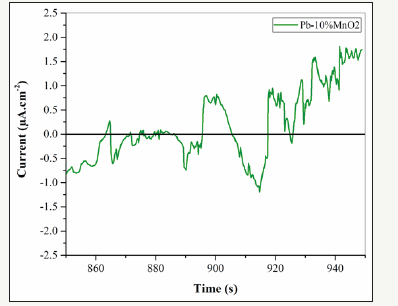Figure 15:The electrochemical noise diagrams for Pb-15MnO2 (wt-%).Figure 16:The electrochemical noise diagrams for Pb-20MnO2 (wt-%).Table 3: Statistical parameters obtain from electrochemical noise analysis.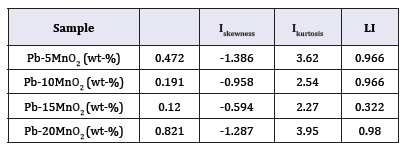In mentioned above equations; x ̅, σi, Iskewness, Ikurtosis and LI are the mean of current densities or potentials, standard deviation, skewness current density, kurtosis current density and localize index respectively. Data calculated from above equations for Pb-5MnO2 (wt-%), Pb-10MnO2 (wt-%), Pb-15MnO2 (wt-%) and Pb-20MnO2 (wt-%) have been illustrated in Table 3. According to ASTM  amounts of noise parameters for the 5, 10 and 20 (wt- %) MnO2 composite coatings have been observed in the range of pitting corrosion. So, it seems that all of these coating anodes have pitting corrosion. LI quantities of these samples show the localized corrosion. Otherwise pb-15MnO2 (wt-%) has shown mixture of uniform and localized corrosion according to its LI. Iskewness and Ikurtosis for pb-15MnO2 (wt-%) indicate almost the uniform corrosion of the surface. If values of x ̅ get more amount the corrosion resistance gets less value. In this study pb-15MnO2 (wt-%) has less x ̅ value and pb-20MnO2 (wt-%) has most value. Therefore pb-15MnO2 (wt-%) has the less corrosion activity and pb-20MnO2 (wt-%) has the most corrosion activity in compare to the others.

#### Conclusion

A. The potentiodynamic polarization diagrams show that the Pb–15MnO2 (wt-%) composite coating has the highest Rp about 857/50 (Ω.cm2), Therefore this sample has shown the less corrosion activity in compare to the others.

B. Simulation of samples measured with electrochemical equivalent circuit showed well fitting with the Bode diagrams.

C. The values of the parameters obtained from the equivalency illustrated the corrosion resistance of Pb-15MnO2 (wt-%) was about 30% higher than the Pb-10MnO2 (wt-%) and 50% higher than the Pb-20MnO2 (wt-%).

D. The Bode diagrams showed that the Pb-15MnO2 (wt-%) coating has better protective properties than the others.

E. The impedance-frequency curve indicated that the Pb- 15MnO2 (wt-%) coating has the lowest failure frequency, and therefore it has been less separation of the substrate than the other coatings during the test process.

Iskewness and Ikurtosis have been obtained from electrochemical Noise study indicate almost the uniform corrosion of the surface for pb-15MnO2 (wt-%).LI, Iskewness and Ikurtosis parameters for Pb-5MnO2 (wt-%), Pb-10MnO2 (wt-%) and Pb-20MnO2 (wt-%) indicated the pitting corrosion mechanisms.

We at Crimson Publishing are a group of people with a combined passion for science and research, who wants to bring to the world a unified platform where all scientific know-how is available read more...